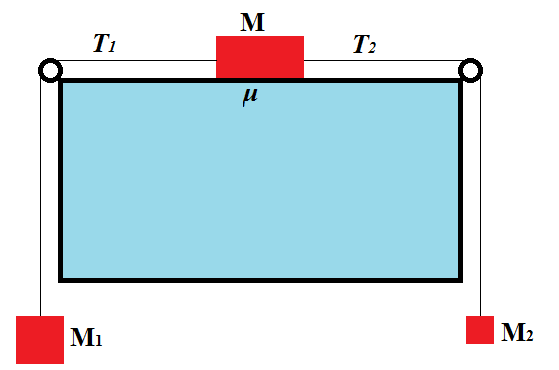## Thursday, June 7, 2018

### Unizor - Physics4Teens - Mechanics - Dynamics - Friction - Problems 3

Notes to a video lecture on http://www.unizor.com

Friction Problems 3

Problem A

An object of mass M can slide on a horizontal table. Two weights of masses M1 and M2 are attached to it on both sides of a table with weightless thread as on this picture.Assuming mass M1 is greater than M2, the object will start sliding to the left under a pull of a bigger weight.

Tensions T1 and T2, as well as friction act on an object on a table.

Assume that this object, as a result of actions of these forces, moves with acceleration a.

What is the friction coefficient μ and magnitudes of tensions T1 and T2?

Hint:

Use the Second Newton's Law for each of three objects (one on a table and two weights) participating in the motion.

Solution:

For object on a table:

T1 − T2 − μ·M·g = M·a

For weight on the left:

M1·g − T1 = M1·a

For weight on the right:

T2 − M2·g = M2·a

Got linear system of three equations with three unknowns: friction coefficient μ and magnitudes of tensions T1 and T2.